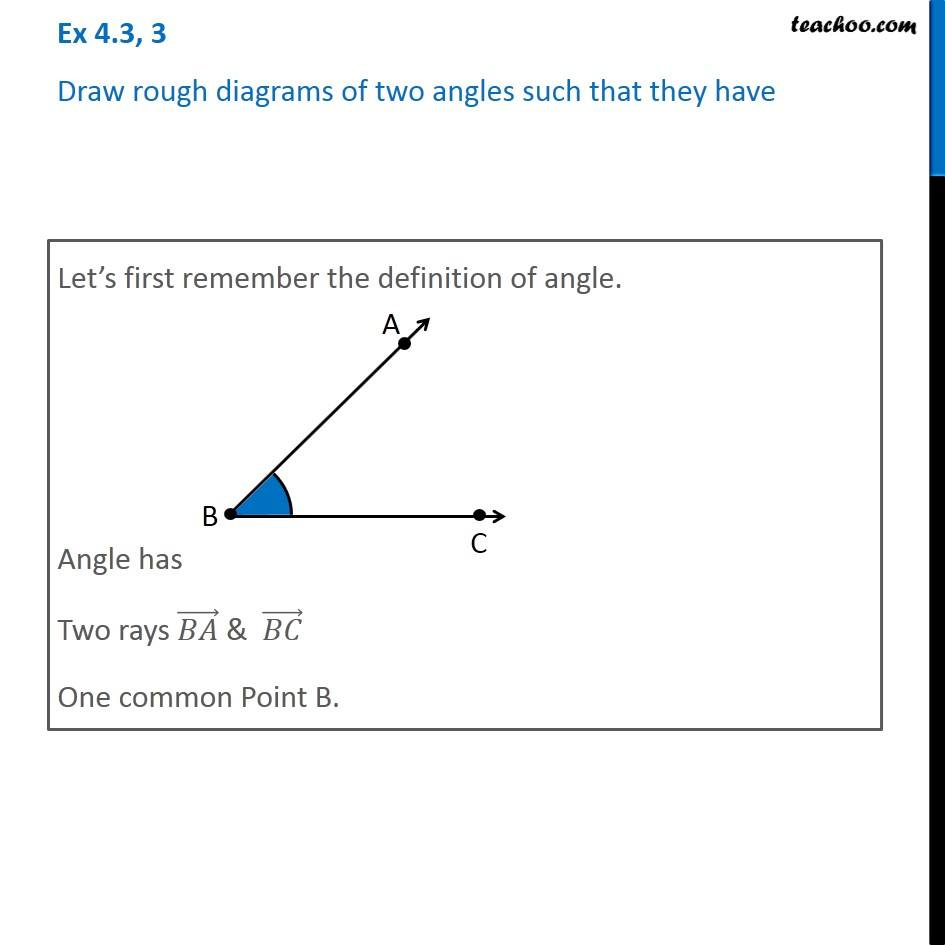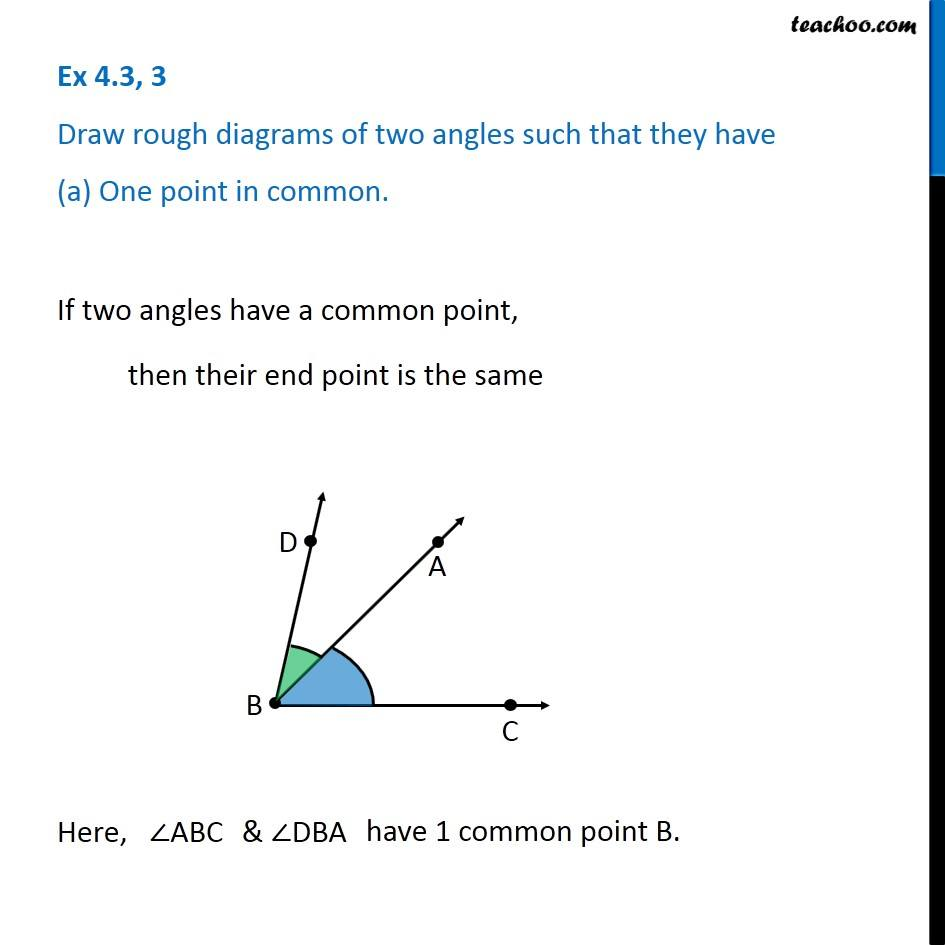Angles

Chapter 4 Class 6 Basic Geometrical Ideas
Concept wiseIntroducing your new favourite teacher - Teachoo Black, at only ₹83 per month

### Transcript

Ex 4.3, 3 Draw rough diagrams of two angles such that they have (a) One point in common. If two angles have a common point, then their end point is the same Here, ABC